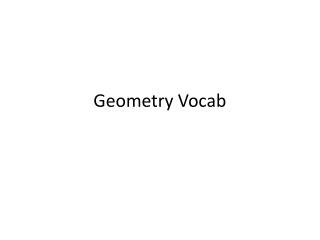DownloadDownload PresentationGeometry Vocab

# Geometry Vocab

Télécharger la présentation## Geometry Vocab

- - - - - - - - - - - - - - - - - - - - - - - - - - - E N D - - - - - - - - - - - - - - - - - - - - - - - - - - -
##### Presentation Transcript

1. Geometry Vocab

2. Inductive reasoning The process of reasoning that a rule or statement is true because specific cases are true

3. Conjecture A statement that is believed to be true

4. Expression A mathematical phrase that contains operations, numbers, and/or variables

5. Constant A value that does not change

6. Difference The result of subtraction

7. Function rule An algebraic expression that defines a function

8. Rate of change A ratio that compares the amount of change in the dependent variable to the amount of change in the independent variable AKA: SLOPE

9. Variable A symbol used to represent a quantity that can change

10. Coefficient A number multiplied by a variable

11. Formula A literal equation that states a rule for a relationship among quantities

12. Equation A mathematical sentence that shows two expressions are equivalent

13. Segment of a line A part of a line consisting of two end points and all points between them

14. Midpoint The point that divides a segment into two congruent segments

15. Congruent Having the same size and shape, denoted by *objects and shapes use congruent

16. Equal Having the same numeric value *measurements and distances use equal

17. Bisect To divide into two congruent parts

18. Proof An argument that uses logic to show that a conclusion is true

19. Postulate A statement that is accepted as true without proof. Also called an axiom.

20. Distance The absolute value of the difference of the coordinates of the points *a measurement, numeric

21. Length The distance between the two endpoints of a segment

22. Construction A method of creating a figure that is considered to be mathematically precise. Figures may be constructed by using a compass and straightedge, geometry software, or paper folding.

23. Intersect To have one or more points in common

24. Conditional statement A statement that can be written in the form “if p, then q,” where p is the hypothesis and q is the conclusion.

25. Hypothesis The part of a conditional statement following the word if.

26. Conclusion The part of a conditional statement following the word then.

27. Inverse operations Operations that undo each other

28. Converse The statement formed by exchanging the hypothesis and the conclusion of a conditional statement

29. Contrapositive The statement formed by both exchanging and negating the hypothesis and conclusion of a conditional statement

30. Truth value A statement can have a truth value of true or false

31. Biconditional statement A statement that can be written in the form “p if and only if q.”

32. Counterexample An example that proves that a conjecture or statement is false

33. Negation The negation of statement p is “not p,” written as .

34. Logically equivalent statements Statements that have the same truth value

35. Deductive reasoning The process of using logic to draw conclusions

36. Law of Syllogism If a, then b. If b, then c. If a, then c. a→b b→c a→c

37. Point An undefined term in geometry, it names a location and has no size.

38. Line An undefined term in geometry , a line is a straight path that has no thickness and extends forever

39. Plane An undefined term in geometry, a flat surface that has no thickness and extends forever

40. Ray An undefined term in geometry , a part of a line that starts at an endpoint and extends forever in one direction

41. Undefined term A basic figure that is not defined in terms of other figures. *point, line, ray and plane

42. Endpoint A point at an end of a segment or the starting point of a ray.

43. Opposite rays Two rays that have a common endpoint and form a line

44. Collinear Points that lie on the same line

45. Coplanar Points that lie in the same plane

46. Non collinear Points that do not lie on the same line

47. Non coplanar Points that do not lie on the same plane

48. Vertex Common point of two rays that form an angle.

49. measure Size of an object (an angle or length)

50. Degrees What we measure angles in